# Selina Concise Mathematics Class 9 ICSE Solutions Solids [Surface Area and Volume of 3-D Solids]

## Selina Concise Mathematics Class 9 ICSE Solutions Solids [Surface Area and Volume of 3-D Solids]

APlusTopper.com provides step by step solutions for Selina Concise Mathematics Class 9 ICSE Solutions Chapter 21 Solids [Surface Area and Volume of 3-D Solids]. You can download the Selina Concise Mathematics ICSE Solutions for Class 9 with Free PDF download option. Selina Publishers Concise Mathematics for Class 9 ICSE Solutions all questions are solved and explained by expert mathematic teachers as per ICSE board guidelines.

Selina ICSE Solutions for Class 9 Maths Chapter 21 Solids [Surface Area and Volume of 3-D Solids]

Exercise 21(A)

Solution 1: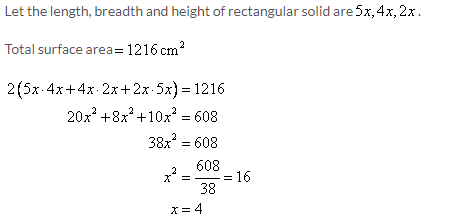Therefore, the length, breadth and height of rectangular solid are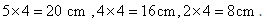Solution 2:Solution 3:Solution 4: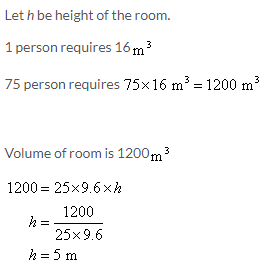Solution 5: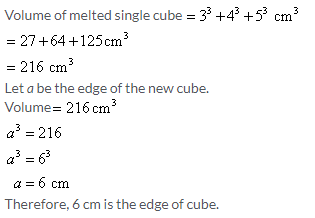Solution 6: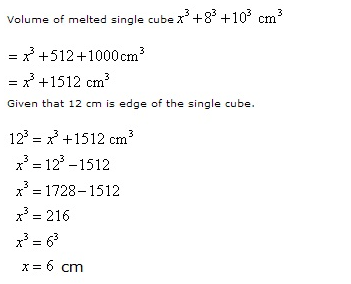Solution 7: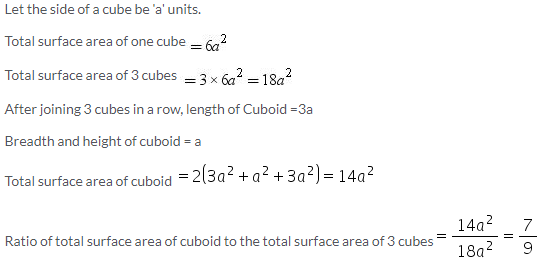Solution 8:Solution 9:
The area of the playground is 3650 m2 and the gravels are 1.2 cm deep. Therefore the total volume to be covered will be:
3650 x 0.012 =43.8 m3.
Since the cost of per cubic meter is Rs. 6.40, therefore the total cost will be:
43.8 x Rs.6.40 = Rs.280.32

Solution 10:Solution 11: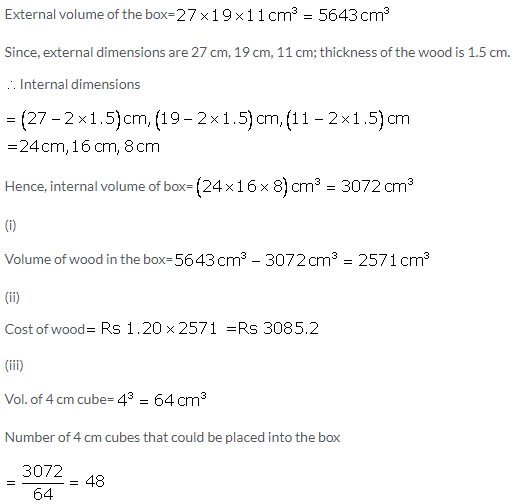Solution 12:Solution 13:
Let exterior height is h cm. Then interior dimensions are 78-3=75, 19-3=16 and h-3 (subtract two thicknesses of wood). Interior volume = 75 x 16 x (h-3) which must = 15 cu dm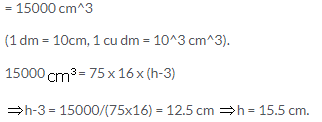Solution 14: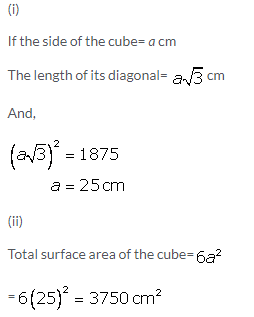Solution 15: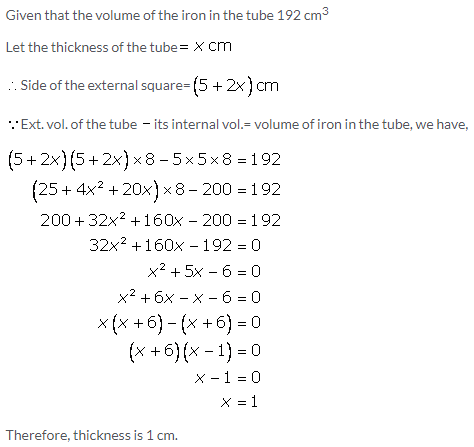Solution 16: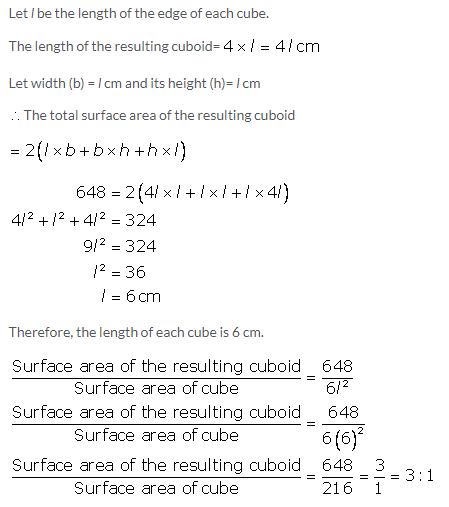Exercise 21(B)

Solution 1:
The given figure can be divided into two cuboids of dimensions 6 cm, 4 cm, 3 cm, and 9 cm respectively. Hence, volume of solid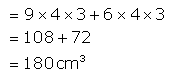Solution 2: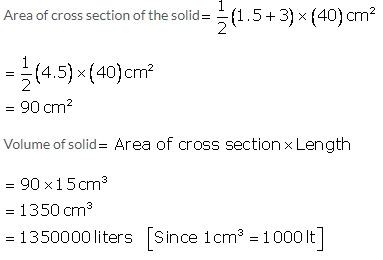Solution 3:
The cross section of a tunnel is of the trapezium shaped ABCD in which AB = 7m, CD = 5m and AM = BN. The height is 2.4 m and its length is 40m.Solution 4:Solution 5: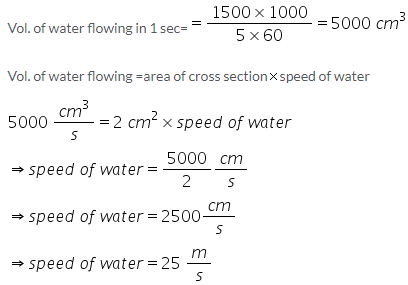Solution 6:Solution 7:Solution 8: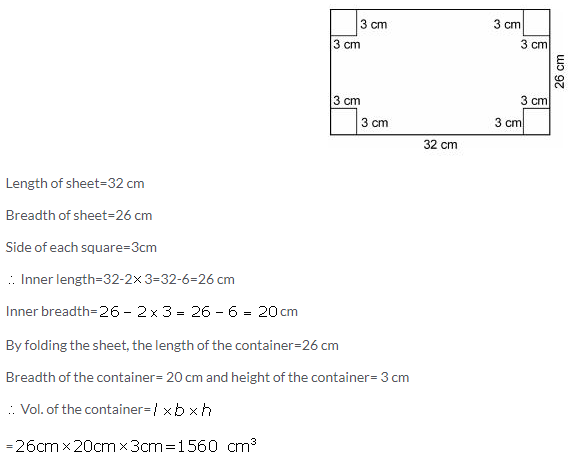Solution 9:Solution 10: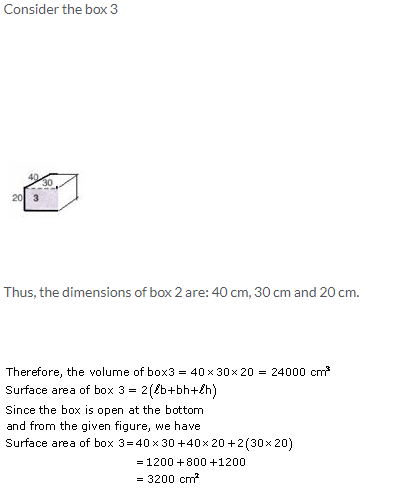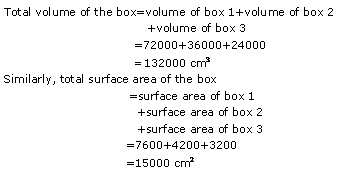Exercise 21(C)

Solution 1: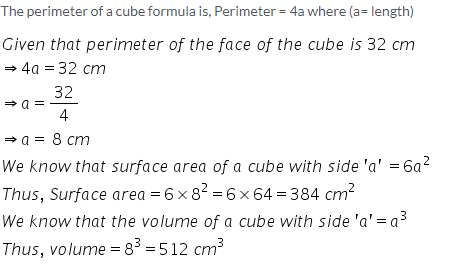Solution 2: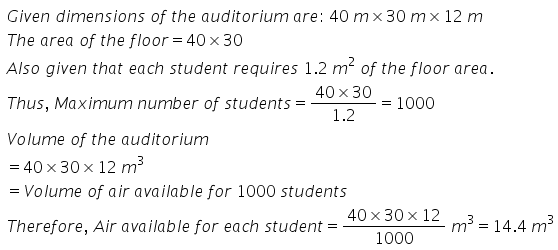Solution 3: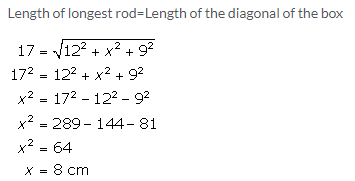Solution 4: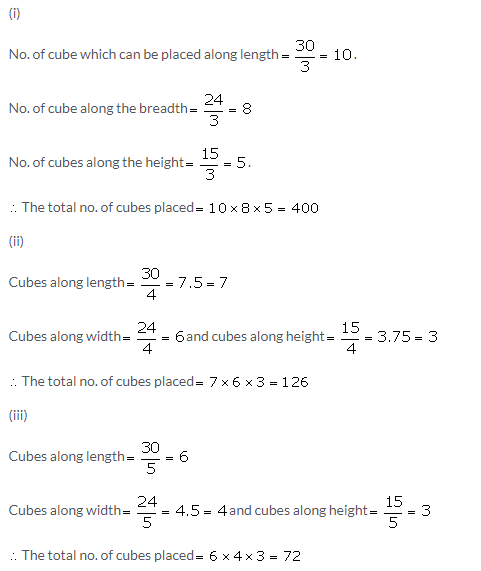Solution 5:Solution 6:Solution 7: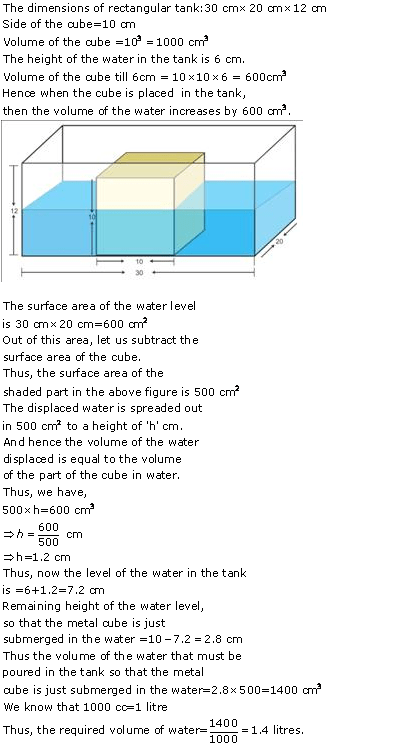Solution 8: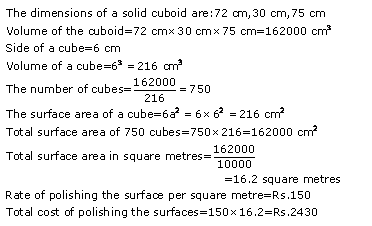Solution 9: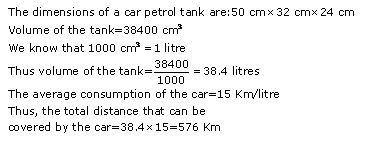Solution 10: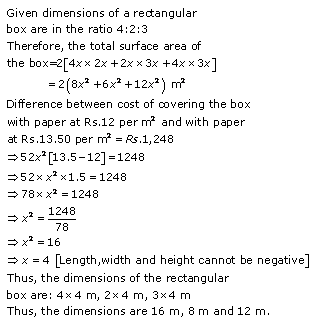More Resources for Selina Concise Class 9 ICSE Solutions